# A Taste of Tangles

Guest post by Tara Fife

This semester, I’ve been doing a reading course with Stefan van Zwam, the bulk of which involved reading interesting papers about tangles. This post highlights some of my favorite ideas so far. We start with an example that essentially comes from Reinhard Diestel and Geoff Whittle’s paper “Tangles and the Mona Lisa” . The goal is to illustrate the intuition behind some of the definitions related to tangles. Precise definitions for connectivity systems and tangles as related to pictures can be found in . Here is a picture of my brother Daniel and me on a ferry outside Seattle.If this picture were printed out and cut into two pieces, then we could sometimes decide that one piece was less important than the other. For instance, if we cut along the red lines below, it is clear that the center piece, while missing some of the beautiful scenery, is the important part of the picture from the perspective of capturing the human subjects of the picture.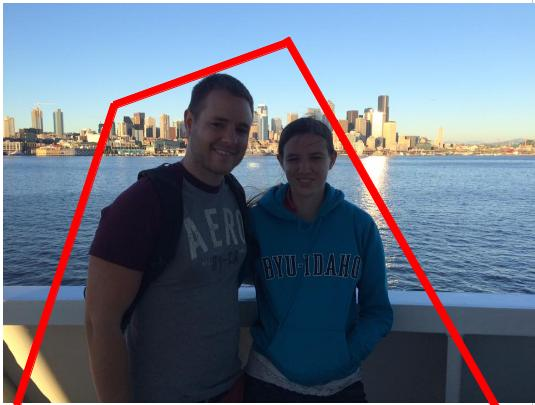If, however, we cut the picture with a squiggly cut, as below, then perhaps neither piece is unimportant. A connectivity system $K=(E(K),\lambda_K)$ consists of a finite set, $E(K)$, together with a connectivity function $\lambda _K:2^{E(K)}\rightarrow\mathbb{R}$ that gives us a way to rank the size of cuts. As such, we expect $\lambda_K(X)=\lambda_K(E(K)-X)$, for all subsets $X$ of $E(K)$. That is, we expect $\lambda_K$ to be symmetric. We also want our connectivity function to be submodular, that is, $\lambda_K(X)+\lambda_K(Y)\geq \lambda_K(X\cap Y)+\lambda_K(X\cup Y)$ for all subsets $X$ and $Y$ of $E(K)$. Any function which is symmetric and submodular is a connectivity function.If $\lambda_1$ and $\lambda_2$ are connectivity functions on the same set, then it is straightforward to check that so is $\lambda_1+\lambda_2$ and, for a positive constant $c$, both $c\cdot\lambda_1$and $\lambda_1+c$. If $K=(E,\lambda_K)$ and $K’=(E,\lambda_{K’})$ are connectivity systems, then we say that $K’$ is a tie breaker if $\lambda_{K’}(X)\leq \lambda_{K’}(Y)$ whenever $\lambda_K(X)\leq \lambda_K(Y)$ and $\lambda_{K’}(X)\not=\lambda_{K’}(Y)$ unless $X=Y$ or $X=E-Y$. Geelen, Gerards, and Whittle’s “Tangles, tree-decompositions and grids in matroids”  proves the following.

Lemma 1 All connectivity functions have tie breakers.

Proof. Let $K=(E,\lambda)$ be a connectivity function. We can assume that $E=\{1, 2, \ldots, n\}$ Define $\lambda_L$ by $\lambda_L(X)=\sum_{i\in X}2^i$ for $X\subseteq {1, 2,\ldots, n-1}$, and $\lambda(X)=\lambda(E-X)$ for $X$ containing $n$. We need to show that $\lambda_L$ is submodular. If $X,Y\subseteq\{1, 2,\ldots, n-1\}$, then
\begin{align*}
\lambda(X)+\lambda(Y) & =\sum_{i\in X}2^i+\sum_{i\in Y}2^i=\sum_{i\in X\cap Y}2^i+\sum_{i\in X-Y}2^i+\sum_{i\in Y}2^i=\sum_{i\in X\cap Y}2^i+\sum_{i\in X\cup Y}2^i\\
& =\lambda_L(X\cap Y)+\lambda_L(X\cup Y).
\end{align*}

If $X\subseteq\{1,2,\ldots,n-1\}$ and $n\in Y$, then
\begin{align*}
\lambda(X)+\lambda(Y) & =\sum_{i\in X}2^i+\sum_{i\not\in Y}2^i=\sum_{i\in X\cap Y}2^i+\sum_{i\in X-Y}2^i+\sum_{i\not\in Y\cup X}2^i+\sum_{i\not\in X-Y}2^i\\
& \geq\sum_{i\in X\cap Y}2^i+\sum_{i\not\in X\cap Y}2^i=\lambda_L(X\cap Y)+\lambda_L(X\cup Y).
\end{align*}

If $n\in X$ and $n\in Y$, then
\begin{align*}
\lambda_L(X)+\lambda_L(Y) & =\lambda_L(E-X)+\lambda_L(E-Y)\\
& =\lambda_L((E-X)\cap(E-Y))+\lambda_L((E-X)\cup(E-Y))\\
& =\lambda_L(E-(X\cup Y))+\lambda_L(E-(X\cap Y)).
\end{align*}

Thus $\lambda_L$ is a connectivity function. Now let $\lambda'(X)=2^n\cdot\lambda(X)+\lambda_L(X)$. It is straightforward to check that $K’=(E,\lambda’)$ is a tie breaker for $K$.

$\square$

A tangle can be thought of as a collection $\mathcal{T}$ of less important (or small ) pieces. So far, we expect that the tangle should only make decisions about relatively simple cuts, and that the tangle should make a decision for all of the simple cuts. If we decide that the two pieces cut out of the picture below are both small, then we don’t want the part of the picture that is left to be contained in a small piece. More precisely, if $K$ is a connectivity system, then a collection $\mathcal{T}$ is a tangle of order $\theta$ in $K$ if

(T1)
$\lambda_K(A) < \theta$, for all $A \in \mathcal{T}$.
(T2)
For each separation $(A,B)$ of order less that $\theta$, either $A\in\mathcal{T}$ or $B\in\mathcal{T}$.
(T3)
If $A,B,C\in\mathcal{T}$, then $A\cup B\cup C \not=E(K)$.
(T4)
$E(K)-e$ is not in $\mathcal{T}$, for each $e\in E(K)$.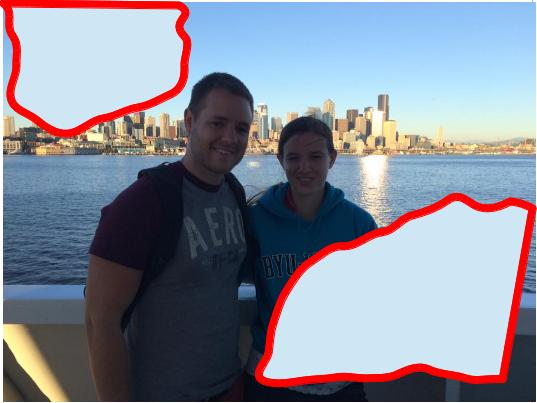For some types of cuts, there might be different opinions about which part is less important. For instance, in the following picture, the matroid community would probably say that the part containing my brother was slightly less important, because the other half contains someone who at least knows the definition of a matroid, while my brother’s company would probably say that the part that contains me is slightly less important.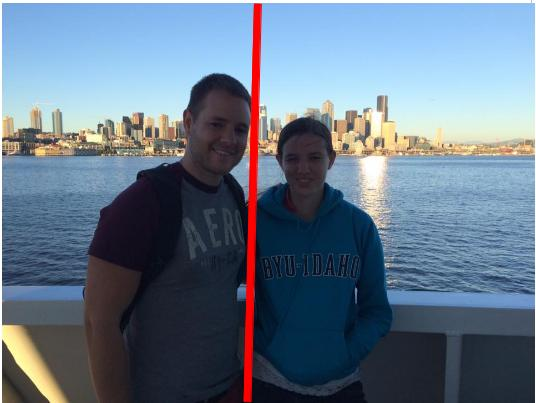My mother would not consider either part unimportant, as we are both somewhat meaningful to her. Since the tangle induced by the opinion of the matroid community disagrees with the one induced by the opinion of Daniel’s company about which half of the separation above is less important, that separation is called a distinguishing separation. Since the tangle induced by my mother’s opinions (that is, a piece is only unimportant if it avoids me and avoids Daniel) is a subset of the matroid community tangle (where a piece is unimportant if it avoids me) and a subset of the company tangle (where a piece in unimportant if it avoids Daniel), it is called a truncation of either of them. That is, $\mathcal{T}’$ is a truncation of $\mathcal{T}$ if $\mathcal{T}’\subseteq\mathcal{T}$. If a tangle is not a proper truncation of another tangle, then it is a maximal tangle.

The above definition of a connectivity function includes the usual connectivity function of a matroid $M$, namely, $\lambda(X)=r(X)+r(E-X)-r(M)$. One of the main results of  gives us information about maximal tangles in a matroid. Before we state the theorem, we need to introduce a bit more notation. If $K=(E,\lambda)$ is a connectivity system, $T$ is a tree, and $\mathcal{P}$ a function from $E$ to $V(T)$, then we say that $(T,\mathcal{P})$ is a tree decomposition of $E$. For a subset $X$ of $V(T)$, we define $\mathcal{P}(X)=\{e:P(e)\in X\}$, and for a subtree $T’$ of $T$, we define $\mathcal{P}(T’)=\mathcal{P}(V(T’))$. We say that a separation $(A,B)$ of $K$ is displayed by $(T,\mathcal{P})$ if $A=\mathcal{P}(T’)$ for some subtree $T’$ of $V(T)$ obtained by deleting an edge of $T$ and letting $T’$ be one of the resulting connected components.The order if a separation $(A,B)$ is $\lambda(A)=\lambda(B)$.

Theorem 1

Let $K$ be a connectivity system, and let $\mathcal{T}_1,\mathcal{T}_2,\ldots,\mathcal{T}_n$ be maximal tangles in $K$. Then there is a tree decomposition $(T,\mathcal{P})$ of $E(K)$ with $V(T)=[n]$ such that the following hold.

1. For each $i\in V(T)$ and $e\in E(T)$, if $T’$ is the component of $T-e$ containing $i$, then $\mathcal{P}(T’)$ is not in $\mathcal{T}_i$.
2. For each pair of distinct vertices $i$ and $j$ of $T$, there is a minimum-order distinguishing separation for $\mathcal{T}_i$ and $\mathcal{T}_j$ displayed by $T$.

Before we sketch a proof of this theorem, we give an example that illustrates the statement of the theorem.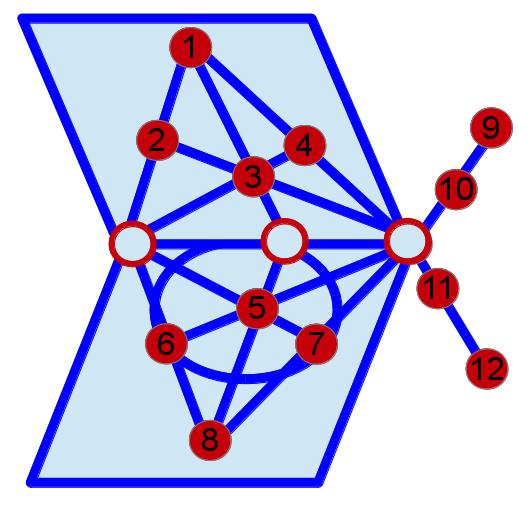Consider the matroid, $M=(,\mathcal{I})$ illustrated above. We first need to determine what the maximal tangles are. Let $S_1=\{9,10\}$, $S_2=\{11,12\}$, and $S_3=$. We will show that an order-1 tangle of $M$ has the form the union of $\{S_i,S_j,(S_i\cup S_j)\}$ together with the set of singletons, where $i$ and $j$ are distinct members of $\{1,2,3\}$.

Let $\mathcal{T}$ be an order-1 tangle of $M$. The sets with connectivity 1 consist of the 12 singletons, their complements, and $S_1$, $S_2$, $S_3$, and their complements. By (T3), at most two of $S_1, S_2, S_3$ are in $\mathcal{T}$. Assume that $\{i,j,k\}=\{1,2,3\}$, and that $S_k$ is not in $\mathcal{T}$. Since $(S_i\cup S_j,S_k)$ is an order-1 separation of $M$, by (T2) $S_i\cup S_j$ is in $\mathcal{T}$. By (T3) (and the fact that there are at least 3 elements of $\mathcal{T}$), we get that neither $S_j\cup S_k$ nor $S_i\cup S_k$ is in $\mathcal{T}$, so by (T2), $S_i$ and $S_j$ are in $\mathcal{T}$.
Since singletons must all be in every tangle of order at least 1, the result follows.

The sets with connectivity 2 either contain $\{1,2,3,4\}$ and avoid $\{5,6,7,8\}$ or contain $\{5,6,7,8\}$ and avoid $\{1,2,3,4\}$. Arguing as above, we get that that the order-2 tangles of $M$ are $\{X:\lambda(X)\leq 2 \text{ and } S\not\subseteq X\}$ for $S\in\{\{1,2,3,4\}, \{5,6,7,8\}\}$. We note that the order-1 tangle where $\{S_i,S_j\}=\{\{1,2,3,4\},\{5,6,7,8\}\}$ is a truncation of both of the order-2 tangles, and that the other order-1 tangles can be represented as $\{X:\lambda(X)\leq 1 \text{ and } \{1,2, 3, 4\}\not\subseteq X\}$ and $\{X:\lambda(X)\leq 1 \text{ and } \{5,6, 7, 8\}\not\subseteq X\}$. Since there are no separations of order-3 or more in $M$, the four maximal tangles of $M$ are $\mathcal{T}_S=\{X:\lambda(X)\leq \lambda(S) \text{ and } S\not\subseteq X\}$, for $S\in\{\{1,2,3,4\},\{5,6,7,8\},\{9,10\},\{11,12\}\}$. For $S \in \{\{1,2,3,4\}, \{9,10\},\{11,12\}\}$, a minimum-order distinguishing separation for $\mathcal{T}_S$ and $\mathcal{T}_{S’}$ is $(S,-S)$, which is displayed by the tree, $T$, below.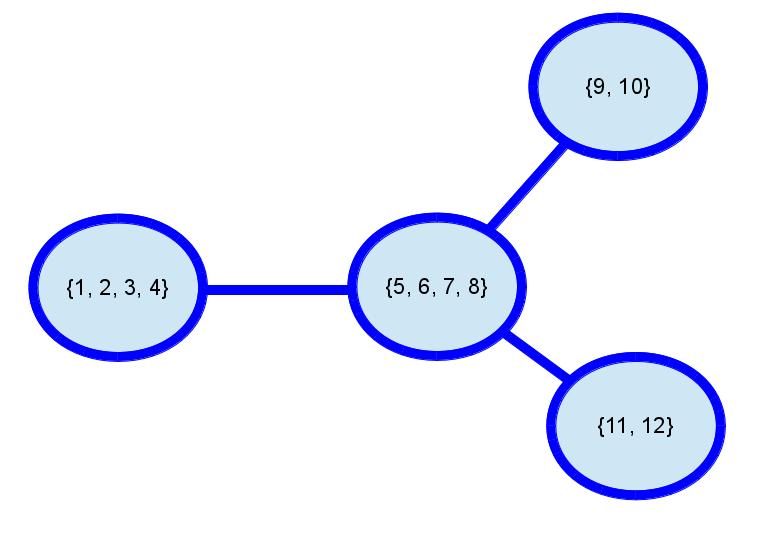The above argument showing that if $S_i\cup S_j$ is in $\mathcal{T}$, then each of $S_i$ and $S_j$ are in $\mathcal{T}$, can be generalized to show the following.

Lemma 2

If $A$ is in a tangle, $\mathcal{T}$ of $K$ of order $\theta$, and $B\subseteq A$ with $\lambda_K(B)\leq\theta$, then $B\in\mathcal{T}$.

To prove Theorem 1, we actually prove the following slightly stronger result also from .

Theorem 2

Let $K$ be a connectivity system, and let $\mathcal{T}_1,\mathcal{T}_2,\ldots,\mathcal{T}_n$ be tangles in $K$, none a truncation of another. Then there is a tree decomposition $(T,\mathcal{P})$ of $E(K)$ with $V(T)=[n]$ such that the following hold.

1. For each $i\in V(T)$ and $e\in E(T)$, if $T’$ is the component of $T-e$ containing $i$, then $\mathcal{P}(T’)$ is not in $\mathcal{T}_i$.
2. For each pair of distinct vertices $i$ and $j$ of $T$, there is a minimum-order distinguishing separation for $\mathcal{T}_i$ and $\mathcal{T}_j$ displayed by $T$.

Proof Sketch
If $K$ is a connectivity system and $K’$ is a tie breaker for $K$, then it is easy to see that a tangle $\mathcal{T}$ of $K$ is also a tangle of $K’$, so it is safe to assume that $K$ is its own tie breaker.

Since $K$ is its own tie breaker, for each distinct $i$ and $j$ in $[n]$, there is a minimum-order separation $(X_{ij},Y_{ij})$ of $K$ distinguishing $\mathcal{T}_i$ and $\mathcal{T}_j$, where $X_{ij}\in\mathcal{T}_i$. Using some well known results (whose statements require terminology which we haven’t introduced), it can be show that there is a tree decomposition $(T,\mathcal{P})$ of $E(K)$ such that each separation $(X_{ij},Y_{ij})$ is displayed by $(T,\mathcal{P})$, that these are the only separations displayed by $(T,\mathcal{P})$, and that each edge of $T$ displays a proper and distinct separation. It remains to show that there is a bijection from $\{\mathcal{T}_1,\ldots,\mathcal{T}_n\}$ to the vertices of $T$ satisfying the conclusion of Theorem 2.

For $i\in[n]$, let $\chi_i$ be the set of subsets $X$ of $V(T)$ such that $E(K)-\mathcal{P}[X]\in\mathcal{T}_i$ and such that $(E(K)-\mathcal{P}[X],\mathcal{P}[X])$ is displayed by $(T,\mathcal{P})$. Each member of $\chi_i$ induces a sub-tree of $T$, and, by (T3), each two members of $\chi_i$ intersect, so the intersection of the members of $\chi_i$ is non-empty. Call this intersection $V_i$. Each edge of $T$ that leaves $V_i$ displays a separation $(A,B)$ with $\mathcal{P}[V_i]\subseteq A$ and $B\in\mathcal{T}_i$. The proof concludes by showing that the $V_i$’s partition the vertices of $T$ into singletons, since this shows that $|V(T)|=n$, and since vertices in $V_i$ satisfy the condition (1) of the theorem.

For each $i\not=j$, the set $\mathcal(V_i)$ is in $Y_{ij}$ and the set $\mathcal(V_j)$ is in $Y_{ji}=X_{ij}$, so the sets $V_1, \ldots, V_n$ are disjoint.

Suppose that $w\in V_i$. We need to show that $\{w\}=V_i$. Choose the edge $e$ incident with $w$ that displays the separation $(X_{ij},Y_{ij})$ of strictly largest order. Since we are assuming that $K$ is its own tie breaker, there is a strictly largest order. Now, each of the other edges incident with $w$ displays a separation of order less than the order displayed by $e$, and hence, less than the orders of $\mathcal{T}_i$ and $\mathcal{T}_j$. Then, by the definition of $(X_{ij},Y_{ij})$, none of these separations distinguish $\mathcal{T}_i$ and $\mathcal{T}_j$. By the definition of $V_i$ and the fact that $X_{ij}$ is in $\mathcal{T}_i$, we know that $\mathcal{P}(w)$ is not a subset of $X_{ij}$ so it is a subset of $Y_{ij}$. Then by Lemma 2, for each of the separations induced by edges other than $e$ incident to $w$, the set not containing $\mathcal{P}(w)$ is in $\mathcal{T}_j$, and hence it is in $\mathcal{T_i}$. Thus, $V_i=\{w\}$.
$\square$

The complete details of the last proof can be found in  along with a corollary which bounds the number of maximal tangles.

As hinted at in the example, tangles can end up pointing to “highly” connected regions of the matroid. This is useful in structure theory because the highly connected regions usually contain the structure that we care about, and a tangle can be used to identify where deletions and contractions can be made while preserving the structure. This idea is utilized, for example, in [3, 4]. Tangles in general grew out of a definition for tangles of graphs, which were used to prove that finite graphs are well quasi ordered.

# References

 R. Diestel, and G. Whittle, Tangles and the Mona Lisa, arXiv:1603.06652v2 [math.CO]

 J. Geelen, B. Gerards, and G. Whittle, Tangles, tree-decompositions and grids in matroids, J. Combin. Theory Ser. B 99 (2009) 657-667

 J. Geelen, and S. van Zwam, Matroid 3-connectivity and branch width, arXiv:1107.3914v2 [math.CO]

 P. Nelson, and S. van Zwam, Matroids representable over fields with a common subfield, arXiv:1401.7040v1 [math.CO]

 N. Robertson and P. D. Seymour, Graph minors, X. Obstructions to tree-decomposition. J. Combin. Theory Ser. B, 52(2):153-190, 1991.